Share

# Some Constructions of Triangles

#### text

Construction: To construct a triangle, given its base, a base angle and sum of other two sides.
Given the base BC, a base angle, say ∠B and the sum AB + AC of the other two sides of a triangle ABC, you are required to construct it.
Steps of Construction :
1. Draw the base BC and at the point B make an angle, say XBC equal to the given angle.
2. Cut a line segment BD equal to AB + AC from the ray BX.
3. Join DC and make an angle DCY equal to ∠BDC.
4. Let CY intersect BX at A (see following Fig.).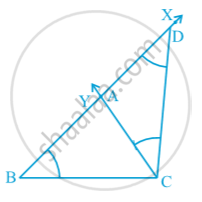Then, ABC is the required triangle.
Let us see how you get the required triangle.
Base BC and ∠B are drawn as given. Next in triangle ACD,
∠ACD = ∠ ADC (By construction)
Therefore, AC = AD and then
AB = BD – AD = BD – AC
AB + AC = BD

Alternative method :
Follow the first two steps as above. Then draw perpendicular bisector PQ of CD to intersect BD at a point A (see following Fig). Join AC. Then ABC is the required triangle. Note that A lies on the perpendicular bisector of CD, therefore AD = AC.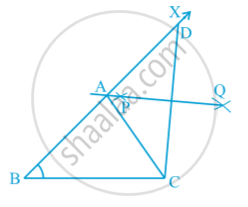Remark : The construction of the triangle is not possible if the sum AB +  AC ≤ BC.

Construction:
To construct a triangle given its base, a base angle and the difference of the other two sides.
Given the base BC, a base angle, say ∠B and the difference of other two sides AB – AC or AC – AB, you have to construct the triangle ABC. Clearly there are following two cases:

Case (i) :
Let AB > AC that is AB – AC is given.
Steps of Construction :
1. Draw the base BC and at point B make an angle say XBC equal to the given angle.
2. Cut the line segment BD equal to AB – AC from ray BX.
3. Join DC and draw the perpendicular bisector, say PQ of DC.
4. Let it intersect BX at a point A. Join AC (see  following Fig.).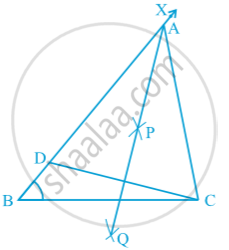Then ABC is the required triangle.
Let us now see how you have obtained the required triangle ABC.
Base BC and ∠B are drawn as given. The point A lies on the perpendicular bisector of DC. Therefore,
So, BD = AB – AD = AB – AC.

Case (ii) : Let AB < AC that is AC – AB is given.
Steps of Construction :
1. Same as in case (i).
2. Cut line segment BD equal to AC – AB from the line BX extended on opposite side of line segment BC.
3. Join DC and draw the perpendicular bisector, say PQ of DC.
4. Let PQ intersect BX at A. Join AC (see following Fig.).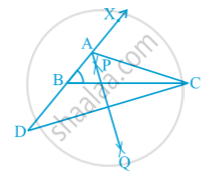Then, ABC is the required triangle.
You can justify the construction as in case (i).

Construction: To construct a triangle, given its perimeter and its two base angles.
Given the base angles, say  ∠ B and ∠ C and BC + CA + AB, you have to construct the triangle ABC.
Steps of Construction :
1. Draw a line segment, say XY equal to BC + CA + AB.
2. Make angles LXY equal to ∠B and MYX equal to ∠C.
3. Bisect ∠ LXY and ∠ MYX. Let these bisectors intersect at a point A (see following fig.)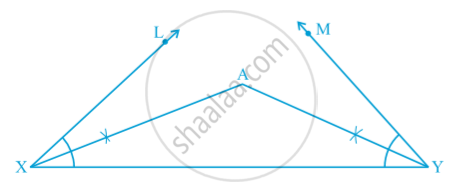4. Draw perpendicular bisectors PQ of AX and RS of AY.
5. Let PQ intersect XY at B and RS intersect XY at C. Join AB and AC [see following Fig].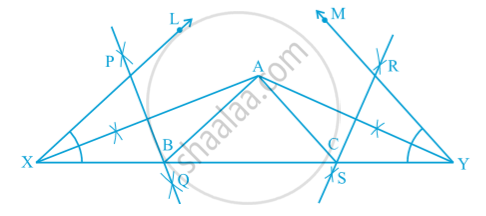Then ABC is the required triangle. For the justification of the construction, you observe that, B lies on the perpendicular bisector PQ of AX. Therefore, XB = AB and similarly, CY = AC. This gives BC + CA + AB = BC + XB + CY = XY.
Again ∠BAX = ∠AXB     (As in ∆ AXB, AB = XB) and
∠ABC = ∠BAX + ∠AXB = 2 ∠AXB = ∠LXY
Similarly, ∠ACB = ∠MYX as required.

### Shaalaa.com

Basic construction part 2 [00:06:20]
S
0%

S